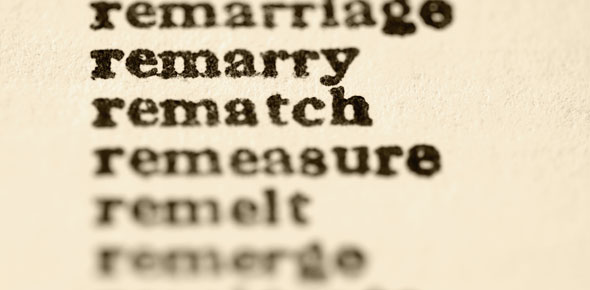# Vocab Quiz Week 4

15 Questions | Attempts: 119
ShareSettings• 1.
1. ............is a rate in which the second quantity in the comparison is one unit.
• 2.
.........is a point at which two or more sides or edges of a geometric figure meet.
• 3.
..........is the horizontal number line in a rectangular coordinate system.
• 4.
..........is the vertical number line in a rectangular coordinate system.
• 5.
........coordinates are the two numbers in an ordered pair, used to identify a location on a map or in a rectangular coordinate system (x,y)
• 6.
........indicates a distance along the x-axis, or horizontal axis, in a rectangular coordinate system (x,_)
• 7.
.......indicates a distance along the y-axis, or vertical axis, in a rectangular coordinate system (_,y)
• 8.
.........is a set of two numbers that identifies a location on a map or in a coordinate system.
• 9.
.......is the point of intersection of the x and y axes, which are number lines that intersect at zero. (0,0)
• 10.
.......is a number that can be written in the form of a common fraction.
• 11.
.......is a number that cannot be written in the form of a common fraction.
• 12.
.......is a kind of drawing that shows mathematical information, ideas, and relationships.
• 13.
.........is the ratio between the picture, or icon, on a graph and the number it represents.
• 14.
......is a series of marks at regular intervals along a line, used for measuring.
• 15.
......represents an actual object but is different in size.

## Related TopicsBack to top
×

Wait!
Here's an interesting quiz for you.